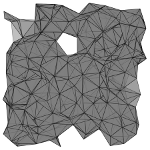# PyDEC: Software and Algorithms for Discretization of Exterior Calculus### Citation

PyDEC: Software and Algorithms for Discretization of Exterior Calculus
Nathan Bell and Anil Hirani
ACM Transactions on Mathematical Software (accepted)

### Abstract

This paper describes the algorithms, features and implementation of PyDEC, a Python library for computations related to the discretization of exterior calculus. PyDEC facilitates inquiry into both physical problems on manifolds as well as purely topological problems on abstract complexes. We describe efficient algorithms for constructing the operators and objects that arise in discrete exterior calculus, lowest order finite element exterior calculus and in related topological problems. Our algorithms are formulated in terms of high-level matrix operations which extend to arbitrary dimension. As a result, our implementations map well to the facilities of numerical libraries such as NumPy and SciPy. The availability of such libraries makes Python suitable for prototyping numerical methods. We demonstrate how PyDEC is used to solve physical and topological problems through several concise examples.